# What Is Algebra Good For In Real Life?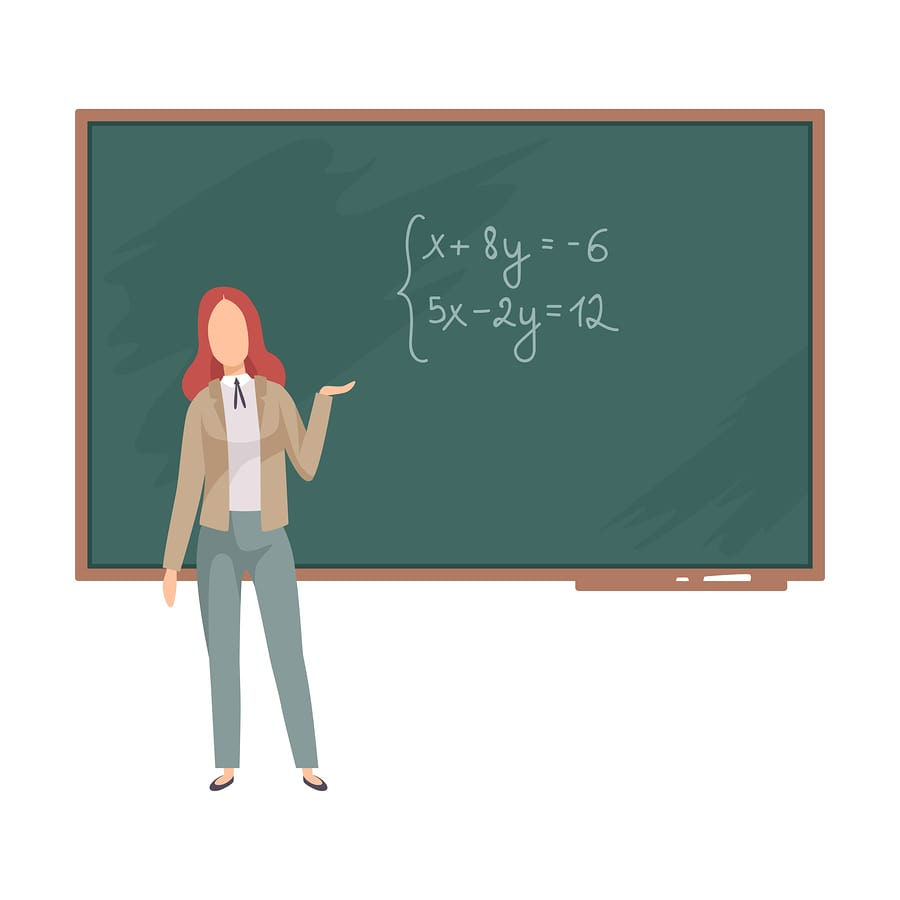## 02 Nov What Is Algebra Good For In Real Life?

Algebra has long been feared by students taking mathematics. Other than the perception of complexity, students also have trouble as they view it as an abstract topic. Without obvious real-life applications, students struggle to make sense of the symbols and equations used.

Yet, algebra at its core was invented as a tool to help us solve problems that existed in the real world. As such, the value of algebra can only be understood by first looking at its origins.

### The History of Algebra

Algebra was first introduced to the world by the Greeks and the Babylonians over 18 centuries ago. In the third century, they devised a system that helped to solve problems with both linear and quadratic equations. In layman terms, it helped them to simplify guess work and to make predictions for everyday tasks.

Guess work has always existed intuitively as people constantly used known variables to make predictions about an unknown variable which it had a relationship with. The difference with algebra was that it replaced numbers with symbols. Instead of having to wrap your head around large ambiguous numbers, simple symbols were used in their place.

### A Simple Example of Algebra’s Value

Instead of stating: “I am finding a number which when multiplied by 8 before having an addition of 2, would give me 50”.

You could now simply rephrase it as “8x + 2 = 50, where x is an unknown number”.

When working with larger sums, complex numbers and convoluted equations, these symbols allow us to simplify the problems for our minds.

### The Application of Algebra

For most students, mention the application of algebra and we think of concepts such as:

• Simultaneous equations
• Differentiation & Integration
• Probability
• Trigonometry
• Binomial theorem

All of these concepts do use algebra to express their various relationships and formulas. This makes algebra an integral part of them. However, these concepts again remain largely abstract in students’ eyes.

To give context of the usefulness of algebra beyond your school, we have collected a list of examples where it is utilised.

### Algebra in Our Everyday Activities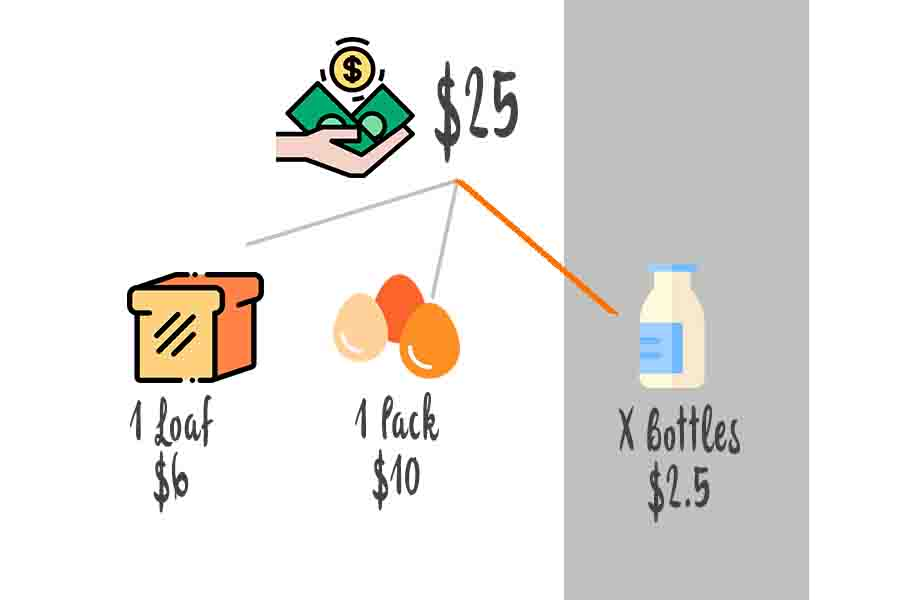Budgeting in a Supermarket

Algebra is intuitively used in daily budgeting. Take the example of a grocery trip to a supermarket.

If you had a budget of \$25 and wanted to buy bread (\$6/loaf), eggs (\$10/pack) & milk (\$2.5/bottle). Assuming the following conditions:

1. You need 1 loaf of bread
2. You need 1 pack of eggs
3. You want as many bottles of milk as possible

A simple equation can be used to determine how much milk to buy: 25 = 6 + 10 + 2.5x, where x is the number of milk bottles. While most people will not explicitly create this equation in their minds, they do intuitively express it in a different form when calculating:

Essentially, you would subtract the cost of bread (\$6) and eggs (\$10) from your max budget (\$25), before dividing the remainder by the cost of a bottle of milk (\$2.5) to find the number of milk bottles (x).

### Algebra in Business & Finance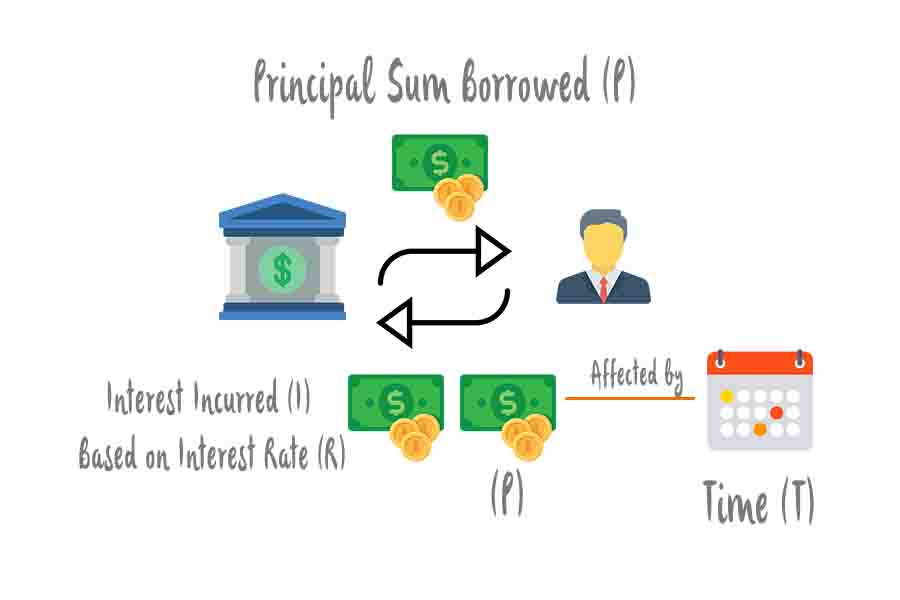Bank Interest on Loans

When you secure a loan from a bank, you will most likely be required to pay back more than the principal borrowed. This is due to the incurring of interest over the duration of the loan. The simple interest amount can be calculated based on the following formula: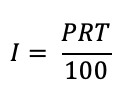I: Simple interest

P: Principal amount borrowed

I: Interest rate, which is set by the bank

T: Duration

Calculating and projecting the simple interest incurred on a loan will help you to determine the duration in which is optimal for you to repay the loan within.

All businesses incur cost in the production of goods which are required in order for a profit to be made from their sale. Through careful planning and forecast, a greater profit can be earned.

The cost and corresponding profit per unit are not constant due to the spreading out of fixed costs among the units produced. As such, it is useful to project the business revenue into the future based on the existing data by using Algebra.

Based on the daily revenue over a period of 1 month, the business owner derives the Revenue formula: R = 11×2 – 500x + 30000

R: Revenue earned for day x

x: exact day of operation

We can forecast the revenue of the subsequent month by the carrying out the following algebra manipulation: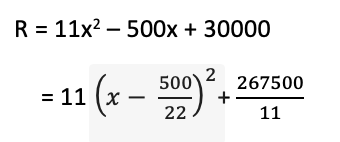From the equation, on day  = 22.7272, the forecasted revenue will be the lowest at  = \$24318.18182. Hence, the business owner will be able to control cost on that specific day by cutting down on the manpower and operating expenses.  This in turn helps to generate the highest profit possible and make sound decision on the business growth and development.

### Algebra in Computer Programming

Our final example of algebra involves an indirect but complex application in the world of computer programming. For clarity, you do not need algebra to perform programming. However, an “algebraic” way of thinking will be of great assistance in coding.

Why do we say that? Programming involves the usage of abstract rules (an equation) to automate the creation of the end result. Within the stated rule, lies various variables which can be expressed as symbols not unlike what we have covered thus far.

Computer programming employs the same type of logic as found in algebra, however since it is intended for machine automation, further rules are added on. These rules can be thought of an expansion of the base formula.

As such, students who are confident in algebra tend to pick up programming logic at a relatively quicker pace.### Einstein’s Takeaway

Algebra is applied intuitively or intentionally to solve a host of different problems in the real world. Even where it is not directly applicable, having trained your mind to be comfortable with algebra, you will find success with many other similar concepts.

In need of a helping hand with mastering algebra at secondary school level? Explore our Sec 3 E Maths Tuition and Sec 3 A Maths Tuition classes. Our lessons are designed to give students a strong foundation in mathematics, giving them the confidence to tackle its many different forms.